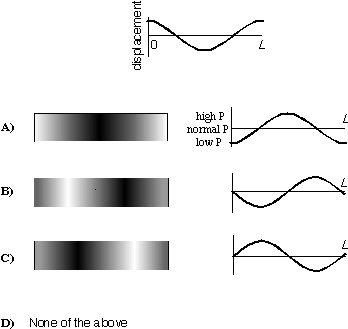TUTORIAL QUESTIONS 152-155

Questions:
152:  Displacement wave at an open end.
153:  Boundary conditions
154:  Pressure wave in a tube open at both ends.
155:  Relationship between travelling and standing waves.

152.  True or False:  The displacement sound wave at the open end of an air tube is always horizontal (i.e., neither rising nor falling), and so the pressure sound wave at the open end of an air tube is always "normal."

a) True
b) False

Fundamentals of Sound reference: Sec. 3-B.

153.  We can apply the same concepts for standing, sinusoidal, transverse waves on strings to standing, sinusoidal, longitudinal waves in air columns, so long as

a) we apply boundary conditions to the displacement wave
b) we apply boundary conditions to the pressure wave
c) we apply boundary conditions to both the displacement wave and the pressure wave
d) we actually can't apply concepts for standing waves on strings to standing waves in air columns.

Fundamentals of Sound reference: Sec. 3-B.

154.  Consider an air tube of length L that is open at both ends and vibrating in its second mode (that is, the second harmonic or first overtone). The displacement wave is shown on the first line of the figure.

Which picture-graph pair below represents the distribution of molecules in the air tube and the pressure wave inside the tube? In the pictures, rarefactions are represented by very light regions and compressions by very dark regions.A)   B)   C   D)

Fundamentals of Sound reference: Secs. 3-A, 3-C.

155.  Any sinusoidal standing wave on a string or air column repeats itself with a period equal to the period of the two traveling waves that make it up.

a) True
b) False

Fundamentals of Sound reference: Sec. 2-A.

Back to Tutorial Listing